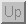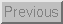Next: Data Acquisition Up: Additional Signal Conditioning Circuits Previous: Comparators

### Signal Energy to Voltage

Motivation

Sometimes, even though a sensor's output is an extremely complex waveform, only the energy of the waveform is needed. This is accomplished by rectifying the waveform (taking the absolute value) and then smoothing (lowpass filtering) the result. This process is shown in the circuit below.Figure 41: Energy to Voltage Conversion

Notice that the output is a rectified version of the input. Figure 42 adds a couple capacitors to the above circuit to smooth the output.Figure 42: A Complete Energy Measurement Circuit

Example - EMG Measurement Using an Electrode

As was discussed previously, the output of the electrode is a voltage waveform that measures underlying neural activity. Many times, when measuring the EMG, the complex waveform that is created by many neural motor units firing is irrelevant. What is of interest is the average energy caused by the muscle exertion. The circuit shown in Figure 42 is used to obtain this energy. The capacitors are usually chosen to smooth off changes faster than 5-10Hz.

Tim Stilson
Thu Oct 17 11:25:23 PDT 1996